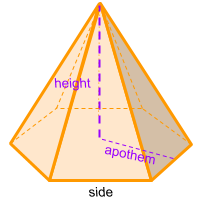Home » Polyhedra » Pyramids » Hexagonal Pyramid

# Volume of a Hexagonal PyramidVolume =
√3/2
× Side ² × Height

## Volume calculator for a hexagonal pyramid

Enter the Side of the hexagon that forms the base of the hexagonal pyramid and enter the height of the pyramid to get the volume automatically.

Another option is to enter the side of the hexagon that forms the base of the hexagonal pyramid and its apothem, and the height of the pyramid to get the volume automatically.

If you already know the area of the base of the pyramid then enter this number along the height of the pyramid to get the volume automatically.

## Description, how many faces, edges and vertices are there in a hexagonal pyramid

The hexagonal pyramid, is a pyramid with a hexagon as base. It has 7 faces, 6 faces are triangles that form the sides and one face considered the base. Which in this case it's a hexagon. It has 12 edges and 7 vertices.

## Formula for the volume of a hexagonal pyramid

To calculate the volume of a hexagonal pyramid, we must first know the area of its base. In other words, we must calculate the area of the hexagon (base). For this we must multiply the perimeter of the hexagon by the apothem and then divide the result by 2.
Once we have the area of the base (area of the hexagon), we multiply it by the height of the pyramid and finally the result is divided by 3.
All of the above is the same as multiplying the length of one side by the apothem and then by the height. In case we don't know the value of the apothem, we can divide the square root of 3 by 2 and then multiply by the length of one side squared and then by the height. You can also use the online calculator to calculate the volume of the hexagonal pyramid automatically.

Volume =
√3/2
× Side ² × Height

Formula explanation and alternative formula:

The formula to calculate the volume of a pyramid is always the same:

(1) Volume pyramid =
Area base × Height / 3

In this case, the area of the base of the pyramid is a hexagon:

Area base = Area hexagon =
Perimeter × Apothem/2

To calculate the perimeter:

Perímetro = 6 × Side

If we replace the area base en the formula (1) we get:

Volume = Side × Apothem × Height

To calculate the apothem:

Apothem =
√3/2
× Side

Therefore, another way to express the formula for the volume of a hexagonal pyramid without knowing the apothem is:

Volume =
√3/2
× Side ² × Height

Share